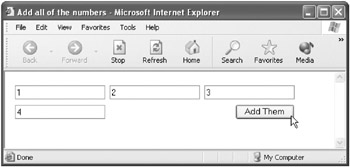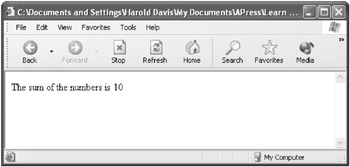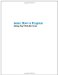# Using a Function s Arguments Array

## Using a Function’s Arguments Array

In the example in the previous section, I showed you how to use a function to add three numbers. Let’s suppose you want to use a function that adds some numbers, but you don’t really know how many numbers you’re going to need to add. It’s easy to do this in JavaScript using the properties of the function.

As I’ll show to you in Chapter 7, “ Working with Objects,” everything in JavaScript is an object. This is also true for most modern programming languages. (We’ll have to leave for Chapter 7 a discussion of the nature of objects.)

Most objects have properties, which are settings related to the object. In the case of a function, one of the properties associated with the function is an array, or list, of the arguments passed to the function. (Chapter 6, “ Programming with Arrays,” explains arrays in detail.)

The array that stores the arguments of a function can be used to access the arguments passed to the function by means of an index mechanism. Furthermore, when you write a function without knowing how many arguments will be passed to it, you can use the length property of the arguments array to find out in your code exactly how many arguments were passed to it. In other words, the length property is a property of the arguments property of a function, and it contains the number of arguments passed to the function. If the function were named addNums, the value contained in the following code:

` addNums.arguments.length `

would tell you the number of arguments passed to the function.

If this sounds a little complicated to you, don’t worry! An example will help. But first, here’s what it boils down to: The function’s arguments array can be used to access arguments passed to the function, even if you don’t know how many arguments to expect. In addition, the function’s arguments.length property will tell you how many arguments were, in fact, passed to the function.

Let’s go over how you would rewrite the addThreeNums function explained in the previous section so that it’ll add however many numbers are passed to it. (The new function is called addNums because it adds any number of numbers!)

First, remove the parameters from the function declaration so that it now appears to not take any arguments:

` function addNums () {  } `

Next, at the top of the function, declare a variable, theAnswer, with a starting value of zero:

` var theAnswer = 0; `

 Tip Because the variable theAnswer has been given a numerical value to start with, the computer knows that it stores a numerical type. If I had used the statement var theAnswer = "0" (note the quote marks), the computer would have known that a string value was being stored. In other words, if you store an unquoted number in a variable in JavaScript, the computer assumes you want to store a numerical type.

The variable theAnswer will be used to return the sum of all the numbers passed to the function.

Next, create a for loop that will be used to cycle through the addNums function’s arguments array (Chapter 4, “ Working with Loops,” explained for loops):

` for (var i = 0; i < addNums.arguments.length; i++) {  } `

 Note The arguments array is zero-based, meaning that the first element in the array has an index of zero and the last element has an index of the length of the array less one. That’s why the for loop starts at zero and keeps iterating as long as the counter variable, i, is less than the number of elements in the arguments array (addNums.arguments.length).

Within the for loop, explicitly convert each argument passed to the array to a number in case it’s actually a text string, as explained in the previous section “ Returns Are Good ”:

` var theNum = Number(addNums.arguments[i]); `

Finally, use the incremental assignment operator (+=) to add the argument to the variable theAnswer that’s being used to store the sum of all the arguments passed to the addNums function.

 Tip The incremental assignment operator (+=) adds the value on the right side of the operator to the variable on the left side and stores the incremented total in the variable on the left side of the operator. In other words, the statement Answer += theNum; is the same as (the longer) Answer = Answer + theNum;.

Once all the arguments have been saved in the variable theAnswer, outside (and beneath) the for loop, return the total stored in the variable:

` return theAnswer; `

Listing 5-4 shows the complete addNums function, which will return the sum of the numbers passed to it, without knowing in advance how many numbers there are to sum. (Of course, the HTML user interface only allows the user to enter three numbers, even though the function will accept more.)

Listing 5.4: Adding Numbers Within a Function Using the Function’s Arguments Array` function addNums () {     var theAnswer = 0;     for (var i = 0; i < addNums.arguments.length; i++) {        var theNum = Number(addNums.arguments[i]);        theAnswer += theNum;     }     return theAnswer;  } `Let’s take this function for a test drive!

I’ve replaced the function used in the HTML page shown in the earlier section “ Returns Are Good ” in Listing 5-3 with the new addNums function, modified the HTML to accept four (rather than three inputs), and modified the onClick event handler of the HTML button to call the new function (see Listing 5-5).

Listing 5.5: Using the addNums Function to Add Four Numbers from an HTML Form (and Display the Results)` <HTML> <HEAD> <TITLE>     Add all of the numbers     </TITLE> <SCRIPT>     function addNums () {        var theAnswer = 0;        for (var i = 0; i < addNums.arguments.length; i++) {           var theNum = Number(addNums.arguments[i]);           theAnswer += theNum;        }        return theAnswer;     }     </SCRIPT> </HEAD> <BODY> <FORM Name="theForm"> <TABLE cellspacing=5> <TR> <TD> <INPUT Type=Text Name="num1"> </TD> <TD> <INPUT Type=Text              Name="num2"> </TD> <TD> <INPUT Type=Text Name="num3"> </TD> </TR> <TR> <TD> </TD> <TD> </TD> <TD align=right> <INPUT Type=Button Value="Add  Them"          onClick='document.write("The sum of the numbers is " +  addThreeNums(theForm.num1.value,theForm.num2.value,theForm.num3.value,              theForm.num4.value));'> </TD> </TR> </TABLE> </FORM> </BODY> </HTML> `If you open the HTML page shown in Listing 5-5 in a Web browser and enter four numbers, it’ll appear as shown in Figure 5-5.Figure 5-5: The function can be used with four boxes (or any number of boxes).

Click Add Them to test the program. The correct sum of the numbers is displayed (see Figure 5-6).Figure 5-6: The sum of the numbers passed to the function is displayed.Learn How to Program Using Any Web Browser
ISBN: 1590591135
EAN: 2147483647
Year: 2006
Pages: 115
Authors: Harold Davis

Similar book on Amazon